# Bieberbach conjecture

(diff) ← Older revision | Latest revision (diff) | Newer revision → (diff)

A hypothesis enunciated in 1916 by L. Bieberbach : For all functions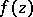of class, i.e. functionswhich are regular and univalent in the discand which have the expansion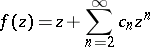in this disc, one has the estimate,, and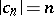only for the Koebe functionswhereis a real number. Bieberbach proved his conjecture for. The problem of finding an accurate estimate of the coefficients for the classis a special case of the coefficient problem.

Owing to its simple formulation and profound significance, Bieberbach's conjecture attracted the attention of numerous mathematicians and stimulated the development of different methods in the geometric theory of functions of a complex variable. At the time of writing (1977) the validity of the Bieberbach conjecture had been established for. It was first proved for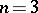in 1923 by K. Loewner who introduced the parametric method (cf. Parametric representation method); other proofs of the estimatesubsequently appeared, and were based on variational methods, on parametric methods and on the method of the extremal metric. The Bieberbach conjecture for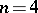was first proved in 1955 by the simultaneous use of variational and parametric methods. In 1960 the estimate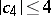was obtained much more simply, with the aid of Grunsky's univalence condition. This estimate was also obtained by variational methods, and with the aid of geometric reasoning; it was once more obtained by using the Grunsky inequalities in matrix form. The validity of Bieberbach's conjecture for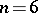was demonstrated in 1968 with the aid of the Grunsky inequalities; it was proved for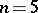in 1972 by variational methods. Among other results obtained in trying to prove the Bieberbach conjecture, the following are worthy of note.

W.K. Hayman  obtained a number of results on the asymptotic behaviour of the coefficients of functions which are-sheeted in, in particular for the class. He proved that the limit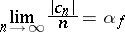exists and that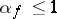with an equality sign only for the Koebe functions.

A number of studies are available on the local Bieberbach conjecture, i.e. the conjecture that the Koebe function gives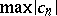at least for those functions in the classwhich are close to it in the relevant topology (cf. Univalent function). It was found that for any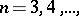there exists a sufficiently smallsuch that for a function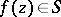which satisfies the condition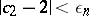the estimateis valid, and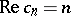only for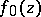.

An estimate of the coefficients for all, accurate as regards the order of dependence on, was first obtained in 1925 by J.E. Littlewood by reducing the estimate for the coefficients to an estimate for the (average) integral of the modulus. More accurate estimates were obtained in 1951 by I.E. Bazilevich (), and in 1965 by I.M. Milin (,).

The best estimates to date (1977) were obtained in 1972 :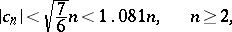and in 1976 :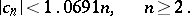For reviews of studies on this subject see , , .

How to Cite This Entry:
Bieberbach conjecture. Encyclopedia of Mathematics. URL: http://encyclopediaofmath.org/index.php?title=Bieberbach_conjecture&oldid=18538
This article was adapted from an original article by E.G. Goluzina (originator), which appeared in Encyclopedia of Mathematics - ISBN 1402006098. See original article